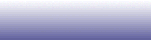Research Home
 RESEARCH Seminars RIT 2020 RIT 2019 RIT 2018 RIT 2014 Analysis 1. Redundancy of Frames Frames are overcomplete sets of vectors in a Hilbert space that satisfy a key norm inequality. They generalize the concept of a spanning set, or a set of generators. Their overcompletness is responsible for providing infinitely many (in general) redundant decompositions of a given vector. While this may be intuitively simple, the concept of redundancy turns out to be very elusive. Some ground work has been made in [8,13,14,18,19,22,27]. There are still open problems, particularly relating various types of geometric decompositions (Feichtinger conjecture) and the Kadison-Singer problem. 2. Localization and Wiener Lemma The intrinsic localization of frames is a property of how the Gramm matrix decays off-diagonal. The faster the decay the better are the geometric properties transferred from finite dimensional frames. The extrinsic localization of frames is obtained by comparing the frame vectors with a reference frame. The reference frames provide additional information which a priorily would be hard to obtain in a direct way. In a simpler formulation, the Wiener lemma states that the reciprocal of an absolutely convergent Fourier series that vanishes nowhere has also an absolutely convergent Fourier series. In the context of frames various extensions of Wiener lemma imply good localization properties (e.g. smoothness and fast decay) of canonical dual frames. Papers [23,26,27] and a recent arXive preprint present some contributions to this issue. Open problems include boundedness of analysis opearators for nonuniform Gabor sets with generators in W(C,l1), and extensions of geometric properties (including the Feichtinger conjecture) to such sets. 3. Reconstruction from magnitudes of frame coefficients Consider the linear transformation y=Ax of an unknown vector x by a linear operator A. If y is measured precisely and the matrix A admits a left inverse, say B, then x=By provides a reconstruction method for x. However there are cases when only partial information about y is measured. One such instance is given by the case when the magnitudes of the entries of y are known. The problem is then to reconstruct x, up to a global ambiguity (a constant phase), from possibly noisy measurements |y|. One approach has two steps (or two phases). In step one one reconstructs a band-diagonal matrix associated to the rank-one operator xx^*. In step two one recovers the signal x by solving an optimization problem. The solution to the first step is connected to the problem of constructing frames for spaces of Hilbert-Schmidt operators. The second step is somewhat more elusive. Due to inherent redundancy in recovering x from its associated rank-one operator, the reconstruction problem allows supplemental conditions. Relevant papers are [17,21,25] and conference papers [39,40]. Applications 1. Signal reconstruction from magnitudes of Fourier transform. Signals of interest are speech signals (reconstruction from spectrograms), image signals (such as X-ray images), and communication signals on fiber optics. A typical signal enhancement (noise reduction) scheme works as follows. First the input signal (be it audio or image) is transformed using e.g. Short-Time Fourier transform; then magnitudes of transformed coefficients are filtered using one of many available filters (Wiener filter, Ephraim-Malah's short-time spectral amplitude MMSE, hard thresholding (a.k.a. spectral subtraction in this context); finally the original noisy phase is applied to transformed magnitudes, and a linear reconstruction algorithm (such as overlap-add in the case of speech signals) is applied to these coefficients to produce the enhanced signal. A more involved processing scheme is used in another relevant problem, nameley the Blind Source Separation problem. Yet a common denominator is the use of linear reconstruction in conjunction with noisy phase. Our problem is to obtain a nonlinear reconstruction scheme (or a nonlinear production model) that uses only spectral magnitudes. 2. Large Scale Optimizations and Sensor Arrays Consider a large scale sensor array having N sensors that monitors a surveillance area. Using all sensors simultaneously may be unreasonable in terms of power consumption and data processing. For example, for N = 10000 sensors and a data sampling rate of 100000 samples per second, the bandwidth requirement is 1Gsamples/sec. We could poll a only subset of D sensors at any one given time instead. The N choose D number of choices of sensors allows for a myriad of sensor configurations, and the task is then to choose a subset that achieves this objective. The approach involves intelligent space-time-frequency sampling as well as large scale optimization problems. 3. Statistical Multimodal Data Modeling and Processing Environment understanding and control combines several components of signal processing with decision optimization all performed in a statistical principled way. For instance inputs from large microphone arrays are processed to monitor and prognostic health status of industrial systems. Similar techniques are used in scene understanding of battle field scenarios. In yet another application, TCP traffic is monitored at several routers for intrusion detection. Signals of interest may have known signatures or they may require learning techniques to extract relevant features. Short Term Projects 1. Intrinsic Dimension Estimation of Natural Images (Summer 2020) The purpose of this project is to estimate several types of dimension of the set of natural images. More Details